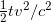## Does "A clock at equator runs more slowly than a clock at the pole" has experiment support?

I don't know if any experiment has been done to check this idea, because my work has nothing to do with Special Relativity.

I believe this idea is based on the wrong reasoning. The following comes from Section 4, Part I of Einstein's paper:

From this there ensues the following peculiar consequence. If at the points A and B of K there are stationary clocks which, viewed in the stationary system, are synchronous; and if the clock at A is moved with the velocity v along the line AB to B, then on its arrival at B the two clocks no longer synchronize, but the clock moved from A to B lags behind the other which has remained at B by(up to magnitudes of fourth and higher order), t being the time occupied in the journey from A to B.

It is at once apparent that this result still holds good if the clock moves from A to B in any polygonal line, and also when the points A and B coincide.

If we assume that the result proved for a polygonal line is also valid for a continuously curved line, we arrive at this result: If one of two synchronous clocks at A is moved in a closed curve with constant velocity until it returns to A, the journey lasting t seconds, then by the clock which has remained at rest the travelled clock on its arrival at A will besecond slow. Thence we conclude that a balance clock at the equator must go more slowly, by a very small amount, than a precisely similar clock situated at one of the poles under otherwise identical conditions.

The conclusion about the clocks is initially got for the case when the two reference frames are relatively moving in the direction of X axis. (Here its correctness is not our concern.) I can not see any reason why this result can be applied to the relative motion in any other directions.

In logic, we can go from a general case to an individual case, but not the reverse. For example, we can say "All students in my class are at least 170cm tall. John is one student in my class. So he must be at least 170cm tall." But we can not say "John is at least 170cm tall. John is one student in my class. So all students in my class are at least 170cm tall."